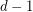# Vizing

## A generalization of Vizing's Theorem? ★★

Author(s): Rosenfeld

Conjecture   Letbe a simple-uniform hypergraph, and assume that every set ofpoints is contained in at mostedges. Then there exists an-edge-coloring so that any two edges which sharevertices have distinct colors.

Keywords: edge-coloring; hypergraph; Vizing